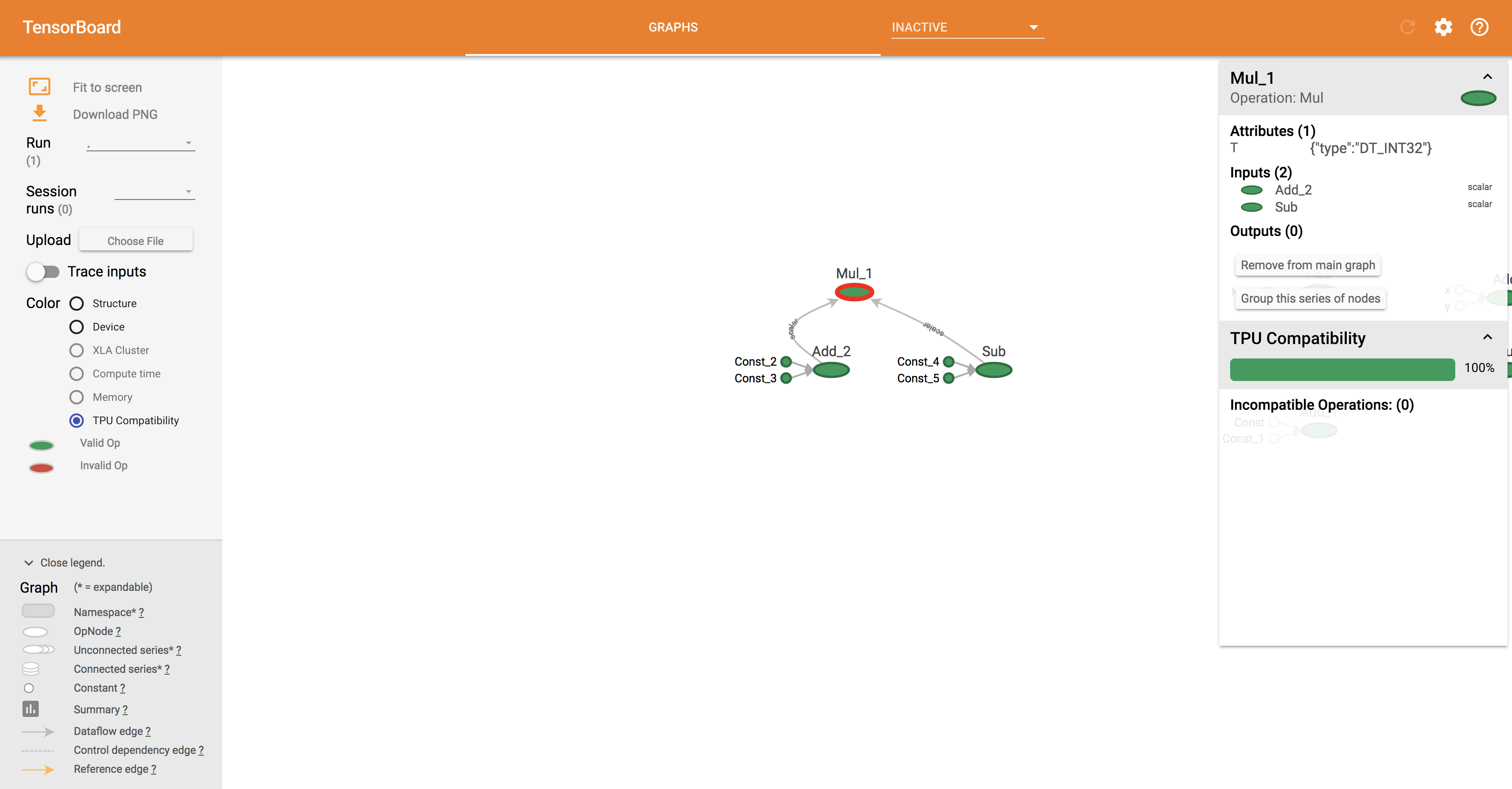## Simple Tensorflow arithmetic

Lets imagine we have to do a simple arithmetic: (10+20) * (30-40)

In some unclear reason we decidet to use Tensorflow.

Default language is there python.

(tensorflow) margusja@IRack:~/tensorflow/tensorflow_scripts\$ python
Python 3.6.0 |Continuum Analytics, Inc.| (default, Dec 23 2016, 13:19:00)
[GCC 4.2.1 Compatible Apple LLVM 6.0 (clang-600.0.57)] on darwin
>>> import tensorflow as tf
/Users/margusja/tensorflow/lib/python3.6/importlib/_bootstrap.py:205: RuntimeWarning: compiletime version 3.5 of module ‘tensorflow.python.framework.fast_tensor_util’ does not match runtime version 3.6
return f(*args, **kwds)
>>>
>>> a = tf.constant(10)
>>> b = tf.constant(20)
>>> c = tf.constant(30)
>>> d = tf.constant(40)
We can explore graph in tensorbar: